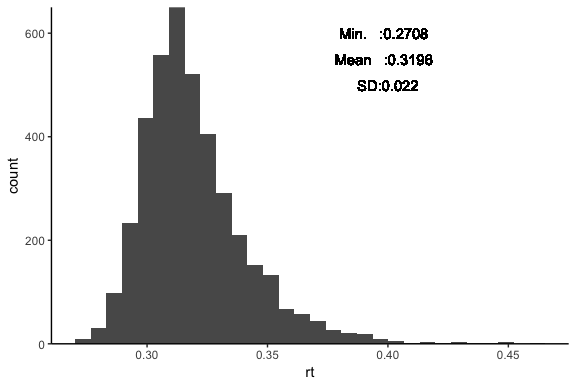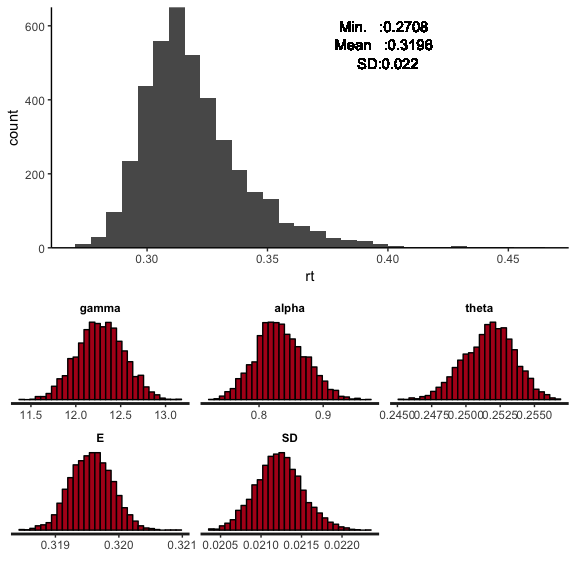# Shifted-Wald Distribution for Response Time Data using R and Stan

## Author Information

Other articles(blog)

## Introduction

The Shifted-Wald distribution(SWD) is propoesed as both a useful measurement tool and intraindividual process model for psychological response time (RT) data( Anders et al., 2016).

Especially, I think the SWD will be useful in some case of minimum RT value(e.g. The shortest time to step on the brake of the car) changes according to independent variables(e.g. age). In this example, the estimated minimum RT can be quantified with θ.

As other examples, individual speed of information accumulation(or processing) will be reflected on gamma parameters. This assumption will deepen the insight of psychological studies. And alpha parameter(response threshold) may be useful for modeling the difficulty of experimental stimulus.

## Shifted-Wald distribution

### probability density function

$f(X|γ, α, θ) \frac{α} {\sqrt{2π(X - θ)^3}} ・exp\biggl\{-\frac{[α-γ(X-θ)]^2}{2(X-θ)}\biggr\}$

• γ parameter affect mass in the tail: speed of the information accumulation

• α affect deviation around the mode, and determine normality of the distribution: threshold parameter

• θ determine the onset of the distribution (location), but not the distribution shape (distribution of mass): non-decision time

The figure 2 and 3 irustrate intuitive and easy to understand the meanings of parameters.See details.

## Stan model

• Shifted-Wald probability density function in Stan(functions block).
• Optionally, Expectation and SD are estimated in transformed parameters block.

• Please save below code as .stan file, “Shifted_Wald.stan”.

functions {
real shifted_Wald_lpdf(real X, real gamma, real alpha, real theta){
real tmp1;
real tmp2;
tmp1 = alpha / (sqrt(2 * pi() * (pow((X - theta), 3))));
tmp2 = exp(-1 * (pow((alpha - gamma * (X-theta)),2)/(2*(X-theta))));
return log(tmp1*tmp2) ;
}
}
data{
int t;
vector<lower=0>[t] RT;
}
parameters {
real<lower=0> gamma;
real<lower=0> alpha;
real<lower=0> theta;
}
transformed parameters{
real<lower=0> E;
real<lower=0> SD;
E = alpha/gamma+theta;
SD = sqrt(alpha / pow(gamma,3));
}
model{
for(i in 1 : t)
target+=shifted_Wald_lpdf(RT[i]|gamma,alpha,theta);
}


## library

#libraries
library(rstan) #rstan
library(gamlss.dist) #including ex-Gaussian
library(tidyverse) #tidy packages
#stan prallel
rstan_options(auto_write = TRUE)
options(mc.cores = parallel::detectCores())

## data preparation

• Generating sample data(random numbers) from ex-gaussian distribution because I don’t know how to make random numbers from SWD.

• In advance, checking the Minimum, Mean and SD value.

#random number from ex-gaussian
df<-data.frame(rt = rexGAUS(4000,mu=10,sigma=1,nu=1/0.5))%>%
dplyr::mutate(rt = rt/100+0.2) #minimum rt will become arround 0.25
summary(df)
##        rt
##  Min.   :0.2708
##  1st Qu.:0.3051
##  Median :0.3155
##  Mean   :0.3196
##  3rd Qu.:0.3296
##  Max.   :0.4595
sd(df$rt) ##  0.02188752 ### visualization sample data #plot gg_rt<-ggplot(df,aes(x=rt))+ geom_histogram()+ theme_classic()+ scale_y_continuous(expand = c(0,0))+ geom_text(mapping = aes(x=0.4,y=600,label = summary(df)))+ geom_text(mapping = aes(x=0.4,y=550,label = summary(df)))+ geom_text(mapping = aes(x=0.4,y=500,label = paste("SD:", round(sd(df$rt),3),sep="")))

#plot
gg_rt## Stan fit and its results

• stan fit
#datastan
datastan = list(RT = df$rt, #RT data t = length(df$rt)) #number of response

#fitting(from .stan file)
fit<-stan(file="Shifted_Wald.stan",data=datastan)
• results
library(formattable)
round(summary(fit)\$summary[,c(1,3,4,8,9,10)],3)%>%
data.frame()%>%
formattable()
mean sd X2.5. X97.5. n_eff Rhat
gamma 12.276 0.260 11.773 12.778 712.109 1.001
alpha 0.834 0.038 0.763 0.912 655.426 1.002
theta 0.252 0.002 0.248 0.255 684.376 1.001
E 0.320 0.000 0.319 0.320 4000.000 1.000
SD 0.021 0.000 0.021 0.022 1420.509 1.000
lp__ 10017.602 1.121 10014.824 10018.924 1381.294 1.000
• results(graphs)
gg_res<-stan_hist(fit)
library(Rmisc)
multiplot(gg_rt,gg_res)# Enjoy!!

### Reference

Anders, R., Alario, F.-X., & Van Maanen, L. (2016). The shifted Wald distribution for response time data analysis. Psychological Methods, 21(3), 309-327. http://dx.doi.org/10.1037/met0000066